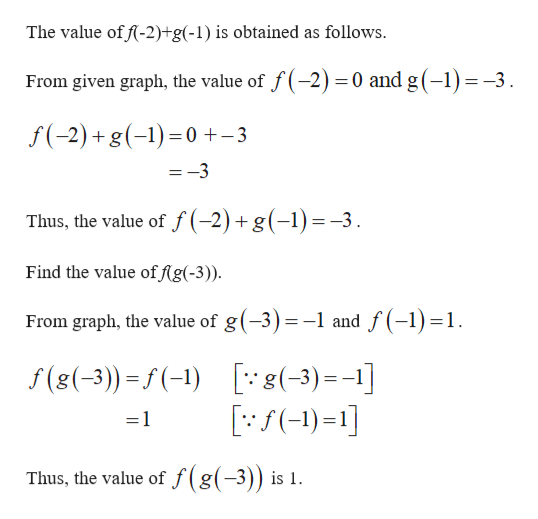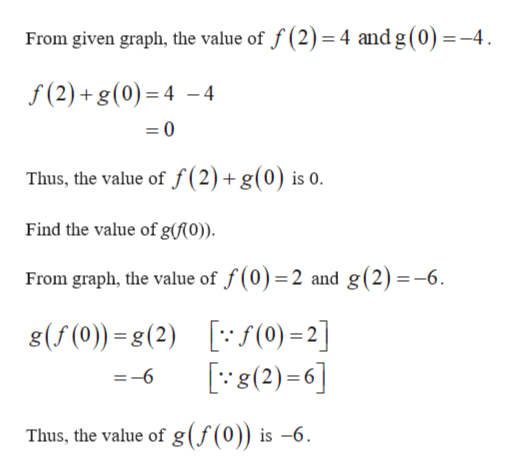Question
1 views

Use the graph of f and g to find the following:

f(-2)+g(-1)

f(g(-3))

f(2)+g(0)

g(f(0))

check_circle

star
star
star
star
star
1 Rating
Step 1

Find the values of f(-2)+g(-1), f(g(-3)), f(2)+g(0), and g(f(0)).help_outlineImage TranscriptioncloseThe value of f-2)+g(-1) is obtained as follows. From given graph, the value of f(-2) 0 and g(-1)-3. f(-2)+g()0+-3 =-3 Thus, the value of f(-2) + g(-1)=-3. Find the value off(g(-3)) From graph, the value of g(-3)=-1 and f(-1)1 [8(-3)=-1] ) f(8(-3) f(-) f(g(3)) is 1 Thus, the value of fullscreen
Step 2

Find the values of f(2)+g(0) and g(...help_outlineImage TranscriptioncloseFrom given graph, the value of f (2)= 4 and g (0)=-4 f(2)+g(0) 44 Thus, the value of f (2)+ g(0) is 0. Find the value of g(f(0)). From graph, the value of f(0)=2 and g(2) =-6 g(f(0))-g(2) f(0) =2] (2)6] =-6 Thus, the value of g (f(0)) is -6 fullscreen

### Want to see the full answer?

See Solution

#### Want to see this answer and more?

Solutions are written by subject experts who are available 24/7. Questions are typically answered within 1 hour.*

See Solution
*Response times may vary by subject and question.
Tagged in

### Math